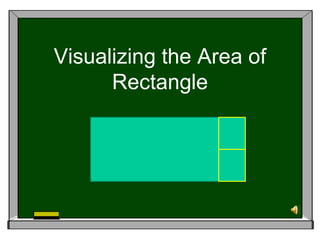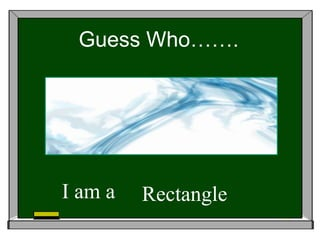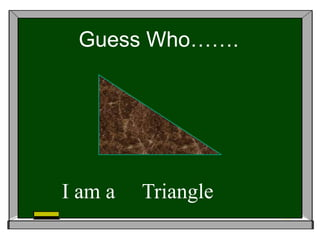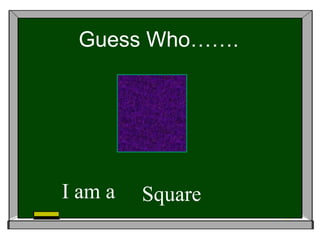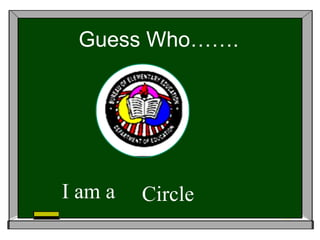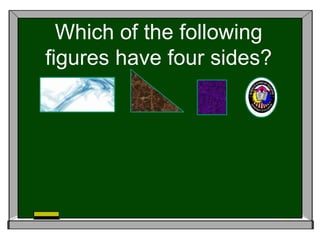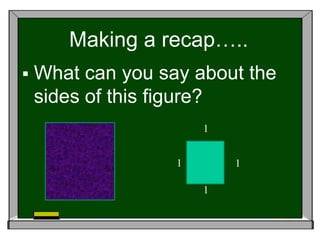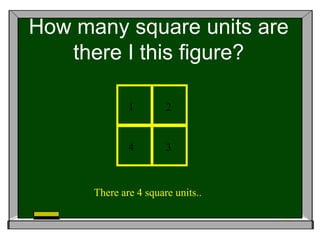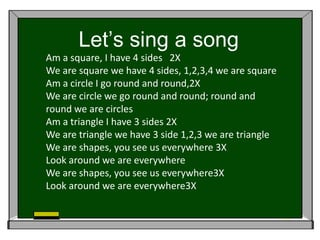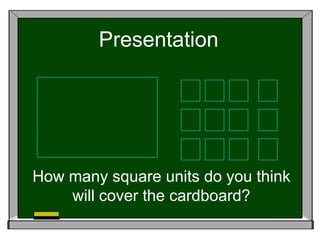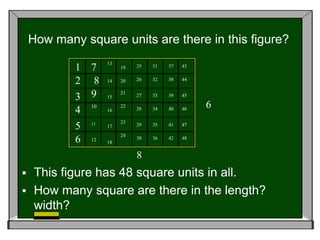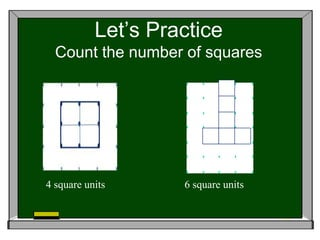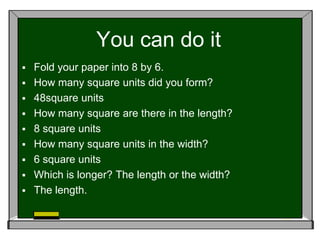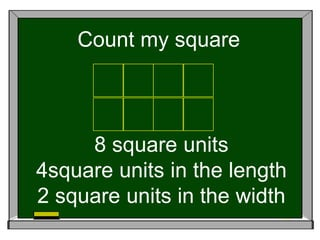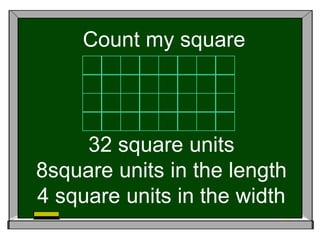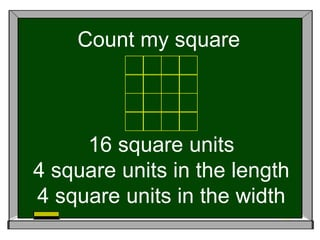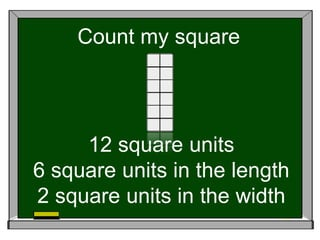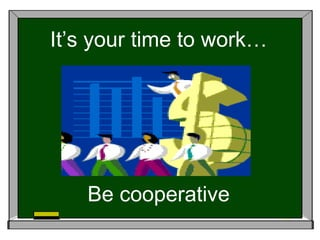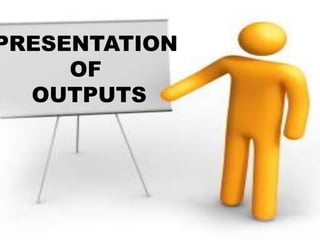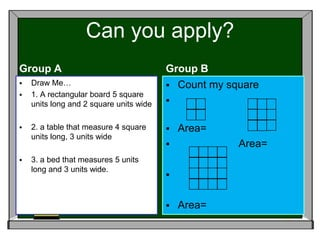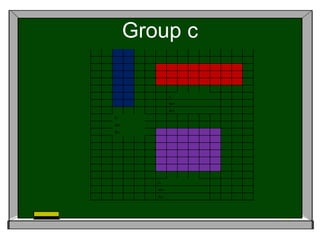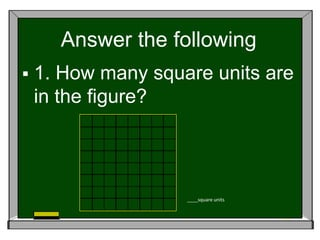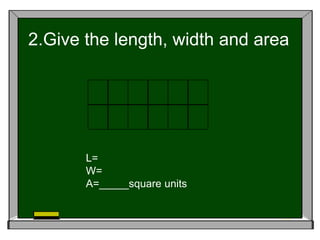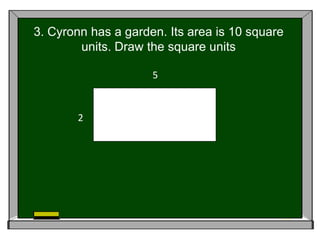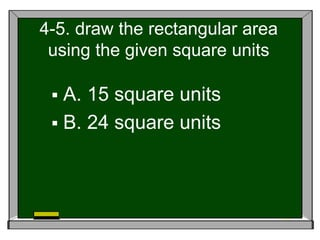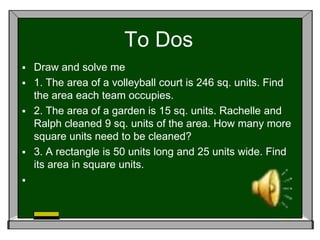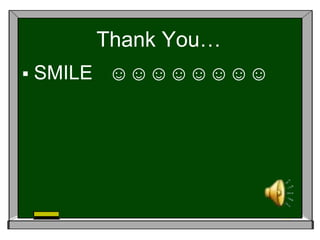1 of 27

### Visualizing the area of rectangle

1. Visualizing the Area of Rectangle
2. Guess Who……. I am a Rectangle
3. Guess Who……. I am a Triangle
4. Guess Who……. I am a Square
5. Guess Who……. I am a Circle
6. Which of the following figures have four sides?
7. Making a recap…..  What can you say about the sides of this figure? 1 1 1 1
8. How many square units are there I this figure? 1 2 4 3 There are 4 square units..
9. Let’s sing a song Am a square, I have 4 sides 2X We are square we have 4 sides, 1,2,3,4 we are square Am a circle I go round and round,2X We are circle we go round and round; round and round we are circles Am a triangle I have 3 sides 2X We are triangle we have 3 side 1,2,3 we are triangle We are shapes, you see us everywhere 3X Look around we are everywhere We are shapes, you see us everywhere3X Look around we are everywhere3X
10. Presentation How many square units do you think will cover the cardboard?
11. How many square units are there in this figure? 13 1 7 19 25 31 37 43 2 8 14 20 26 32 38 44 3 9 21 15 27 33 39 45 22 6 4 10 16 28 34 40 46 23 5 11 17 29 35 41 47 24 6 12 18 30 36 42 48 8  This figure has 48 square units in all.  How many square are there in the length? width?
12. Let’s Practice Count the number of squares 4 square units 6 square units
13. You can do it  Fold your paper into 8 by 6.  How many square units did you form?  48square units  How many square are there in the length?  8 square units  How many square units in the width?  6 square units  Which is longer? The length or the width?  The length.
14. Count my square 8 square units 4square units in the length 2 square units in the width
15. Count my square 32 square units 8square units in the length 4 square units in the width
16. Count my square 16 square units 4 square units in the length 4 square units in the width
17. Count my square 12 square units 6 square units in the length 2 square units in the width
18. It’s your time to work… Be cooperative
19. PRESENTATION OF OUTPUTS
20. Can you apply? Group A Group B  Draw Me…  Count my square  1. A rectangular board 5 square units long and 2 square units wide   2. a table that measure 4 square  Area= units long, 3 units wide  Area=  3. a bed that measures 5 units long and 3 units wide.   Area=
21. Group c l= w= A= l= w= A= l= w= A=
22. Answer the following  1. How many square units are in the figure? ____square units
23. 2.Give the length, width and area L= W= A=_____square units
24. 3. Cyronn has a garden. Its area is 10 square units. Draw the square units 5 2
25. 4-5. draw the rectangular area using the given square units  A. 15 square units  B. 24 square units
26. To Dos  Draw and solve me  1. The area of a volleyball court is 246 sq. units. Find the area each team occupies.  2. The area of a garden is 15 sq. units. Rachelle and Ralph cleaned 9 sq. units of the area. How many more square units need to be cleaned?  3. A rectangle is 50 units long and 25 units wide. Find its area in square units. 
27. Thank You…  SMILE ☺☺☺☺☺☺☺☺